Friday, March 24, 2023

# Reflection of Light Selina 9th Concise Physics Solutions

Reflection of Light Selina 9th Concise Physics Solutions  Chapter-7 Reflection of Light .Step By Step Revised Concise Selina Physics Solutions of Chapter-7 Reflection of Light with Exe-7(A) , MCQ 7(A),Num 7(A) Exe-7(B) ,  MCQ -7(B) Num 7(B), Exe-7(C),  MCQ-7(C), Num 7(C)for Class-9.  Visit official Website CISCE for detail information about ICSE Board Class-9.

 Board ICSE Publications Selina Publication Subject Physics Class 9th Chapter-7 Reflection of Light Exe-7(A) Book Name Concise Topics Solution of Exercise-7(A),  MCQ-7(A),  Num-7(A),    Exercise-7(A),  MCQ-7(A),  Num-7(A)   and  Exercise-7(A),  MCQ-7(A),  Num-7(A) Academic Session 2021-2022

## Reflection of Light Selina Concise Physics Solutions Class 9th

–: Select Topics :–

Exercise-7(A),  MCQ-7(A),  Num-7(A)

Exercise-7(B),  MCQ-7(B),  Num-7(B)

Exercise-7(C),  MCQ-7(C),  Num-7(C)

Note :-  Before Viewing Selina Concise Physics Solutions of Chapter-7 Reflection of Light for ICSE Class-9 Physics. Read the whole chapter carefully and Solved all example of Chapter-7 Reflection of Light for Class-9 Physics.

Latest syllabus of council for class 9th physics, Law of reflection, character of image, Spherical Mirror, Ray diagram and use of spherical mirror, Type of mirror, radius of curvature, Pole, Principal Axis, Focus and Focal Length.

### Exe-7(A) Reflection of Light Selina Concise 9th physics solutions

#### Question 1

What do you mean by reflection of light?

The return of light into the same medium after striking a surface is called reflection.

#### Question 2

State which surface of a plane mirror reflects most of the light incident on it: the front smooth surface or the back silvered surface.

Black silvered surface reflects most of the light incident on it.

#### Question 3

Explain the following terms:

(a) Plane mirror,  (b) Incident ray,

(c) Reflected ray,  (d) Angle of incidence and

(e) Angle of reflection.

Draw diagram/diagrams to show them.

(a) Plane mirror: Plane mirror is a highly polished and smooth reflecting surface made from a clear plane glass sheet, usually thin and silvered with suitable reflecting abrasive (for example, mercury) on one side. Once this pasting is done, then the glass becomes opaque but due to the reflecting property of the abrasive, the plane glass sheet becomes a plane glass reflector or a plane glass mirror.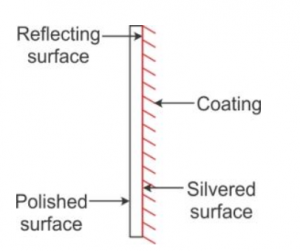(b) Incident ray: The light ray striking a reflecting surface is called the incident ray.

(c) Reflected ray: The light ray obtained after reflection from the surface, in the same medium in which the incident ray is travelling, is called the reflected ray.

(d) Angle of incidence: The angle which the incident ray makes with the normal at the point of incidence is called the angle of incidence. It is denoted by the letter i.

(e) Angle of reflection: The angle which the reflected ray makes with the normal at the point of incidence is called the angle of reflection. It is denoted by the letter r.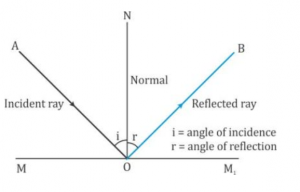#### Question 4

With the help of diagrams, explain the difference between the regular and irregular reflection.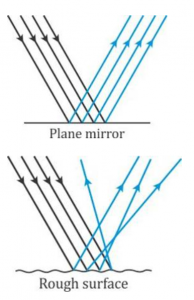Regular reflection occurs when a beam of light falls on a smooth and polished surface and irregular reflection occurs when a beam of light falls on a rough surface. Since the surface is uneven, from different points light rays get reflected in different directions and give rise to irregular reflection.

#### Question 5

Differentiate between the reflection of light from a plane mirror and that from a plane sheet of paper.

Reflection of light from a plane mirror is regular reflection and reflection of light from plane sheet of paper is irregular reflection of light.

#### Question 6

State the two laws of reflection of light.

Laws of reflection:

1. The angle of incidence is equal to the angle of reflection.
2. The incident ray, the reflected ray and the normal at the point of incidence, lie in the same plane

#### Question 7

State the law of reflection and describe an experiment to verify them.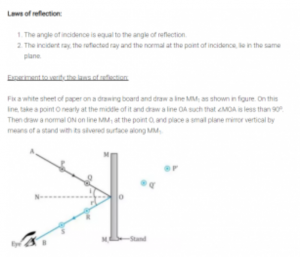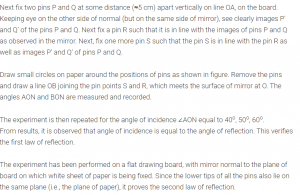#### Question 8

A light ray is incident normally on a plane mirror. (a) What is its angle of incidence?

(b) What is the direction of reflected ray? Show it on a diagram.

(a) 0o

(b) Same as the incident ray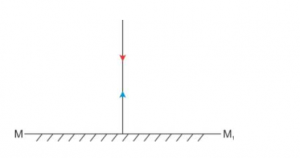#### Question 9

Draw a diagram to show the reflection of a ray of light using a plane mirror. In the diagram, label the incident ray, the reflected ray, the normal, the angle of incidence and the angle of reflection.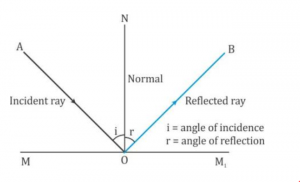#### Question 10

Fig. 7.15 shows an incident ray AO and the normal ON on a plane mirror. The angle which the incident ray AO makes with mirror is 30. (a) Find the angle of incidence. (b) Draw the reflected ray and then find the angle between the incident and reflected rays.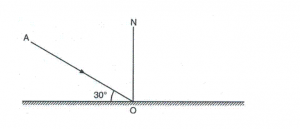(a) Angle of incidence = 90– 30o = 60o

(b) Angle between the incident ray and reflected ray = Angle of incidence + Angle of reflection

Angle of reflection = Angle of incidence = 60o

Therefore, Angle between the incident ray and reflected ray = 60o + 60o = 120o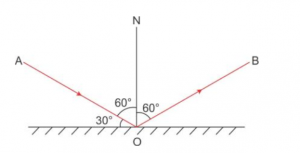#### Question 11

The diagram in Fig. 7.16 shows a point object in front of a plane mirror MM1.

(a) Complete the diagram by taking two rays from the point to show the formation of its image.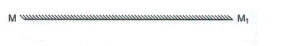(b) In the diagram, mark the position of eye to see the image.

(c) Is the image formed real or virtual? Explain why?

(a) and (b)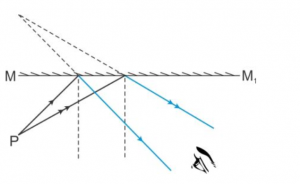(c) The image formed is virtual because the reflected rays meet when produced backwards.

#### Question 12

The diagram below in Fig7.17. shows an object XY in front of a plane mirror. Draw on the diagram, the image of the object formed by the mirror. Trace the path of two rays from a point on the object to show the formation of image.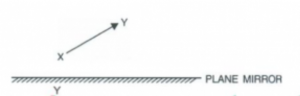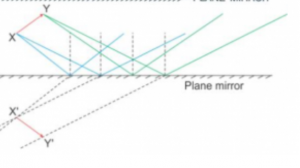#### Question 13

1. a) Write three characteristics of the image formed by a plane mirror?

(b) How is the position of an image related to the position of the object?

(a) Three characteristics of image formed by plane mirror:

(i) Image formed in erect (upright)

(ii) Image formed is virtual

(iii) Image formed is of the same size as the object

(b) The image is situated at the same perpendicular distance behind the mirror as the object in front of it.

#### Question 14

Differentiate between a real and a virtual image.

 Real Image Virtual image 1. A real image is formed due to actual intersection of the reflected rays. 1. A virtual image is formed when the reflected rays meet if they are produced backwards. 2. A real image can be obtained on a screen. 2. A virtual image cannot be obtained on a screen. 3. A real image is inverted with respect to the object. 3. A virtual image is erect with respect to the object.

#### Question 15

What is meant by lateral inversion of an image in a plane mirror? Explain it with the help of a ray diagram.

The interchange of the left and right sides in the image of an object in a plane mirror is called lateral inversion.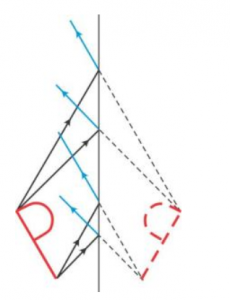Figure above shows the image formation of a letter P in a plane mirror. The letter P appears in the plane mirror as .

#### Question 16

The letters on the front of an ambulance are written laterally inverted like . Give reason.

The letters on the front of a ambulance are written laterally inverted, so that the driver of the vehicle moving ahead of the ambulance reads these words laterally inverted as AMBULANCE, in his rear view mirror, and gices side to pass the ambulance first.

#### Question 17

Why is it difficult to read the image of the text of a page formed due to reflection by a plane mirror?

Due to lateral inversion, , it becomes difficult to read the image of the text of a page formed due to reflection by a plane mirror.

### MCQ-7(A) Reflection of Light Selina  Concise physics solutions

#### Question 1

According to the law of reflection:

(a) i/r = constant

(b) sin i /sin r = constant

(c) = constant

(d) r

r

#### Question 2

The image formed by a plane mirror has the following characteristic:

(a) Erect and diminished

(b) Erect and enlarged

(c) Inverted and of same size

(d) Erect and of same size.

Erect and of same size

#### Question 3

The image formed by a plane mirror is :

(a) real

(b) virtual

(c) virtual with lateral inversion

(d) real with lateral inversion

virtual with lateral inversion

### NUM-7(A) Reflection of Light Selina Concise  physics solutions

#### Question 1

The  ray  is incident on a plane mirror. Its reflected ray is perpendicular to the incident ray.  Find the angle of incidence.

Angle of incidence (i) + Angle of reflection(r) = 90

But, as per the laws of reflection, i = r

Therefore, 2 i = 90o

Or, i = r = 45o

#### Question 2

A man standing in front of a plane mirror finds his image at a distance 6 metre from himself. What is the distance of man from the mirror?

Distance between man and his image = 6m

Distance between man and mirror + distance between mirror and image = 6m

But, Distance between man and mirror (object distance) = distance between mirror and image (image distance)

Therefore, distance of man from mirror = 6/2 = 3m

#### Question 3

An insect is sitting in front of a plane mirror at a distance 1 m from it.

(a) Where is the image of the insect formed? (b) What is the distance between the insect and its image?

(a) Image of the insect is formed 1m behind the mirror.

(b) Distance between the insect and his image = 1 + 1 = 2 m

#### Question 4

An object is kept at 60 cm in front of a plane mirror. If the mirror is now moved 25 cm away from the object, how does the image shift from its previous position?

Initially, distance of the object from the mirror = 60 cm.

Therefore, image is formed at a distance 60 cm from the mirror, behind it.

Thus, initial distance between the object and image = 60 + 60 = 120 cm

If the mirror is moved 25 cm away from the object,

The new distance of the object from the mirror = 60 + 25 = 85 cm

The new image is now at a distance 85 cm from the mirror behind it.

Thus, new distance of the image from the object = 85 + 85 = 170 cm

Taking the position of the object as reference point, the distance between the two positions of the image = new distance of image from the object – initial distance of the image from the object

= (170 – 120) cm = 50 cm

Thus, the image shifts 50 cm away.

#### Question 5

An optician while testing the eyes of a patient keeps a chart of letters 3 m behind the patient and asks him to see the letters on the image of chart formed in a plane mirror kept at distance 2 m in front of him. How far away is the chart seen by the patient?

Distance between man and chart = 3m

Distance between man and mirror = 2m

Therefore, distance between chart and mirror = 5 m

Now, final image is formed on the mirror, which is at a distance of 2 m from the man, therefore, the chart as seen by patient is (5m + 2m =) 7m away.

### EXE-7(B) Reflection of Light Selina Revised Concise 9th physics solution

#### Question 1

Two plane mirrors are placed making an angle in between them. Write an expression for the number of images formed of an object placed in between the mirrors. State the condition, if any.

If two mirrors make an angle  with each other and object is placed in between the two mirrors, the number of images formed is n or (n – 1) depending upon n = 360o /o is odd or even.

(a) If n = 360o /o is odd,

(i) The number of images formed is n, when the object is placed asymmetrically between the mirrors.

(ii) The number of images formed is n-1, when the object is placed symmetrically between the mirrors.

(b) If n = 360o /o is even, the number of images is always n-1.

#### Question 2

Two plane mirrors are placed making an angle θ° in between them. For an object placed in between the mirrors, if angle is gradually increased from 0 to 180, how will the number of images change: increase, decrease or remain unchanged?

The number of images formed is given asSo, if q is gradually increased, n decreases.

#### Question 3

How many images are formed for a point object kept in between two plane mirrors M1 and M2 at right angles to each other? Show them by drawing a ray diagram.

For two mirrors kept perpendicular to each other, three images are formed for an object kept in between them.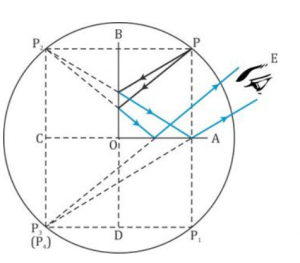#### Question 4

Two plane mirrors are arranged parallel and facing each other at some separation. How many images are formed for a point object kept in between them? Show the formation of images with the help of a ray diagram

For two mirrors kept parallel to each other, an infinite number of images are formed for an object kept in between them.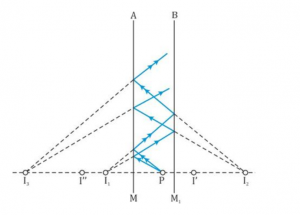#### Question 5

State two uses of a plane mirror.

Two uses of plane mirror:

1. In barber’s shop for seeing the hairs at the back of head, two mirrors facing each other are fixed on opposite walls at the front and back of the viewer.
2. In solar heating devices such as a solar cooker, solar water heater, etc., a plane mirror is used to reflect the incident light rays from sun on the substance to be heated.

### MCQ-7(B) Selina Concise 9th physics solution Reflection of Light

#### Question 1

Two plane mirrors are placed making an angle 60in between them. For an object placed in between the mirrors, the number of images formed will be :

(a) 3  (b) 6

(c) 5  (d) infinite.

5

#### Question 2

In the barber’s shop, two plane mirrors are placed:

(a) Perpendicular to earth other

(b) Parallel to each other

(c) At an angle 60°between them

(d) At angle 45°between them

In a barber’s shop, two plane mirrors are placed parallel to each other.

### Num-7(B) Reflection of Light Selina 9th physics solutions

#### Question 1

State the number of images of an object placed between two mirrors, formed in each case when mirrors are inclined to each other at (a) 90°, and (b) 60°.

(a) Angle between the mirrors,= 90o

Now, n = 360o /o = 360o / 90= 4, which is even.

Hence number of images formed will be (n-1); i.e., 4-1 = 3 images

(b) Angle between the mirrors,= 60o

Now, n = 360o /o = 360o / 60= 6, which is even.

Hence number of images formed will be (n-1); i.e., 6-1 = 5 images

#### Question 2

An object is placed (i) asymmetrically (ii) symmetrically, between two plane mirrors inclined at an angle of 50. Find the number of images formed.

Angle between the mirrors,= 50o

Now, n = 360o /o = 360o / 50= 7.27, which is odd.

(i) When placed asymmetrically, number of images formed will be n, i.e. 7.

(ii) When placed symmetrically, number of images formed will be (n-1); i.e. 7-1 = 6 images

### EXE-7(C) Concise Selina 9th Physics Solutions Reflection of Light

#### Question 1

What is a spherical mirror?

A reflecting surface which is a part of a sphere is called a spherical mirror.

#### Question 2

Name the two kinds of spherical mirrors and distinguish between them.

Two kinds of spherical mirrors are concave and convex.

Distinction between concave and convex mirror: A concave mirror’s bulging surface is silvered and reflection takes place from the hollow surface but a convex mirror’s inner surface is silvered and reflection takes place from the bulging surface.

#### Question 3

Define the terms pole, principal axis and centre of curvature with reference to a spherical mirror.

Pole: The geometric centre of the spherical surface of mirror is called the pole of mirror.

Principal axis: It is the straight line joining the pole of the mirror to its centre of curvature.

Centre of curvature: The centre of curvature of a mirror is the centre of the sphere of which the mirror is a part.

#### Question 4

Draw suitable diagrams to illustrate the action of (i) concave mirror and (ii) convex mirror on a beam of light incident parallel to the principal axis.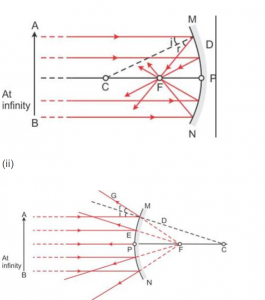#### Question 5

Name the spherical mirror which (i) diverges (ii) converges the beam of light incident on it. Justify your answer by drawing a ray diagram in each case.

(i) Convex mirror diverges a beam of light falling on it.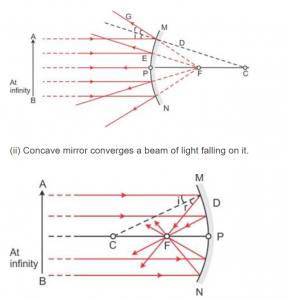#### Question 6

Define the terms focus and focal length of a concave mirror. Draw diagram to illustrate your answer.

Focus of a concave mirror: The focus of a concave mirror is a point on the principal axis through which the light rays incident parallel to principal axis, pass after reflection from the mirror.

Focal length of a concave mirror: The distance of the focus from the pole of the concave mirror is called its focal length.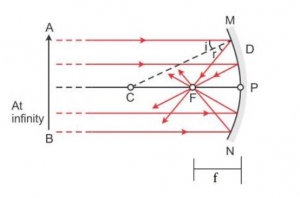#### Question 7

Explain the meaning of the terms focus and focal length in case of a convex mirror, with the help of suitable ray diagram.

Focus of a convex mirror: The focus of a convex mirror is a point on the principal axis from which, the light rays incident parallel to principal axis, appear to come, after reflection from the mirror.

Focal length of a convex mirror: The distance of the focus from the pole of the convex mirror is called its focal length.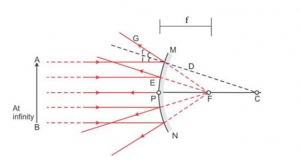#### Question 8

State the direction of incident ray which after reflection from a spherical mirror retraces its path. Give a reason to your answer.

Incident ray is directed towards the centre of curvature because the ray is normal to the spherical mirror, soi =r = O.

#### Question 9

(i) Name the mirrors shown in Fig. 7.49 (a) and (b).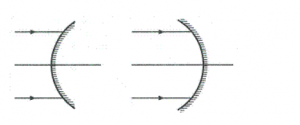(ii) In each case (a) and (b), draw reflected rays for the given incident rays and mark focus by the symbol F.

(a) (b)

(i) In figure (a) a convex mirror is shown.

In figure (b) a concave mirror is shown. (ii) (a)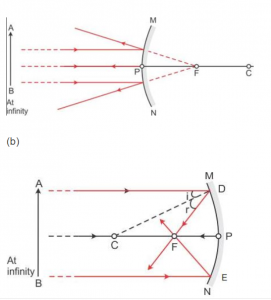#### Question 10

Complete the following diagrams in Fig.7.50  by drawing the reflected rays for the incident rays 1 and 2.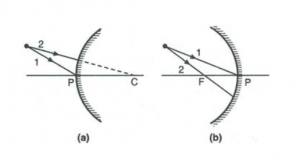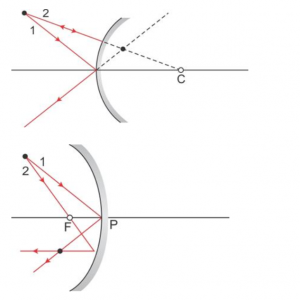#### Question 11

Complete the following diagrams shown in Fig. 7.51 by drawing the reflected ray for each of the incident ray A and B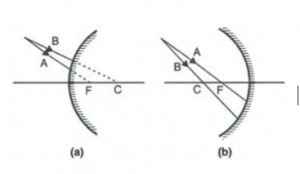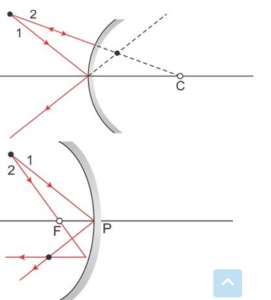#### Question 12

State the two convenient rays that are chosen to construct the image by a spherical mirror for a given object? Explain your answer with the help of suitable ray diagrams.

Two convenient rays that are chosen to construct the image by a spherical mirror for a given object:

1. A ray passing through the centre of curvature:A ray of light passing through the centre of curvature of a concave mirror or a ray directed in the direction of centre of curvature of a convex mirror is reflected back along the same path after reflection.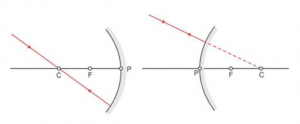1. A ray parallel to the principal axis:A ray of light parallel to the principal axis, after reflection pass through the principal focus in case of a concave mirror or appears to diverge from it in case of convex mirror.#### Question 13

Fig. 7.52 shows a concave mirror with its pole at P, focus and centre of curvature C. Draw ray diagram to show the formation of image of an object OA.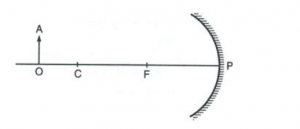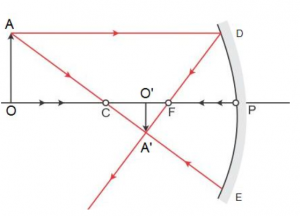#### Question 14

Fig. 7.53 shows a concave mirror with its pole at P, focus and centre of curvature C. Draw ray diagram to show the formation of image of an object OA.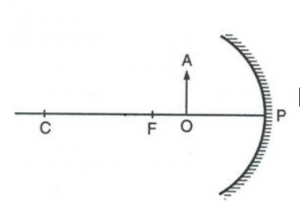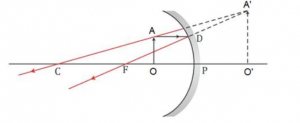#### Question 15

The diagram below in Fig.7.54, shows a convex mirror. C is its centre of curvature and F is its focus. (i) Draw two rays from A and hence locate the position of image of object OA. Label the image IB. (ii) State three characteristics of the image.

The ray diagram shows two light rays from A.

The image of the object OA is formed between the focus and the pole on the other side of the mirror.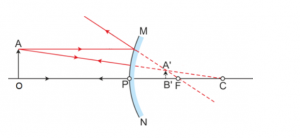The image so formed is erect, virtual and diminished.

#### Question 16

Draw a ray diagram to show the formation of image by a concave mirror for an object placed between its pole and focus. State three characteristics of the image.

Ray diagram showing the formation of an image by a concave mirror for an object placed between its pole and focus: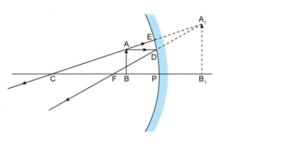When the object is placed between focus F and pole P, the image is formed behind the mirror.

The image so formed is virtual, upright and magnified.

#### Question 17

Draw a ray diagram to show the formation of image by a concave mirror for the object beyond its centre of curvature. State three characteristics of the image.

Ray diagram showing the formation of an image by a concave mirror for the object beyond its centre of curvature: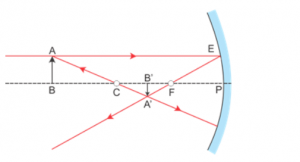When the object is placed beyond the centre of curvature C, the image is formed between focus F and centre of curvature C. The image so formed is real, inverted and diminished.

#### Question 18

Draw a ray diagram to show the formation of image of an object kept in front of a convex mirror. State three characteristics of the image.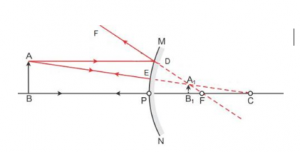The image formed is virtual, erect and diminished.

#### Question 19

Name the mirror which always produces an erect and virtual image. How is the size of image related to the size of object?

Convex mirror always produces erect and virtual images. The images formed are diminished, i.e. the size of the image is shorter than the size of the object.

#### Question 20

(a) For what position of object, the image formed by a concave mirror is magnified and erect?

(b) State whether the image in part (a) is real or virtual?

(a) If the object is placed between the pole and focus of a concave mirror, the image formed is magnified and erect.

(b) The image is virtual.

#### Question 21

(a) State the position of object for which the image formed by a concave mirror is of same size.

(b) Write two more characteristics of the image.

(a) If the object is placed at the centre of curvature of a concave mirror, the image formed is of same size.

(b) The image formed is real and inverted.

#### Question 22

(a) What is a real image?

(b) What type of mirror can be used to obtain a real image of an object?

(c) Does the mirror mentioned in part (b) form real image for all locations of the object?

(a) An image which can be obtained on a screen is called a real image.

(b) A concave mirror can be used to obtain a real image of an object.

(c) No, it does not form real image for all locations of the object.

#### Question 23

Discuss the position and nature of image formed by a concave mirror when an object is moved from infinity towards the pole of mirror.

When an object is moved from infinity towards the pole of mirror, the image formed moves away from the mirror. The image formed is real and inverted.

#### Question 24

Discuss the position and nature of image formed by a convex mirror when an object is moved from infinity towards the pole of mirror.

In a convex mirror, the image formed is always virtual, upright and diminished. It is always situated between its pole and focus, irrespective of the distance of object in front of the mirror.

#### Question 25

Name the kind of mirror used to obtain:

(a) A real and enlarged image,

(b) A virtual and enlarged image,

(c) A virtual and diminished image,

(d) A real and diminished image.

(a) Concave, (b) Concave, (c) Convex and (d) Concave

#### Question 26

How is the focal length of a spherical mirror related to its radius of curvature?

Focal length is half the radius of curvature of a spherical mirror.#### Question 27

Write the spherical mirror’s formula and explain the meaning of each symbol used in it.

The spherical mirror’s formula isHere, u is the object distance, v is the image distance and f is the focal length of the mirror.

#### Question 28

What is meant by magnification? Write its expression. What is its sign for the (a) real (b) virtual, image?

Magnification is the ratio of the length of image to the length of the object.It is also given asWhere, u and v is the object and image distance, respectively.

Hence, we have

(a) For real image: u and v are negative. So, m is negative.

(b) For virtual image: u is negative and v is positive. So, m is positive.

#### Question 29

At what maximum distance the image in a convex mirror can be obtained? What will be the location of object then?

The image formed by a convex mirror is always between pole and focus. Hence, the maximum distance that can be obtained in convex mirror is the focal length. For this case the object has to be at infinity.

#### Question 30

Upto  what maximum distance from a concave mirror, the image can be obtained? What will be the location of object for it?

The maximum distance that can be obtained in concave mirror is infinity. For this case the object has to be at focus.

#### Question 31

How will you distinguish between a plane mirror, a concave mirror and a convex mirror, without touching them?

To distinguish between a plane mirror, concave mirror and convex mirror, the given mirror is held near the face and image is seen. There can be following three cases:

Case (i): If the image is upright, of same size and it does not change in size by moving the mirror towards or away from the face, the mirror is plane.

(ii) If the image is upright and magnified, and increases in size on moving the mirror away, the mirror is concave.

(iii) If the image is upright and diminished and decreases in size on moving the mirror away, the mirror is convex.

#### Question 32

State two uses of a concave mirror.

Two uses of concave mirror:

(i) It is used as a shaving mirror.

(ii) It is used as reflector in torch, head light of automobiles etc.

#### Question 33

State the kind of mirror used

(a) By a dentist,

(b) As a search-light reflector.

(a) Concave mirror

(b) Concave mirror

#### Question 34

(a) When a concave mirror is used as a shaving mirror, where is the person’s face in relation to the focus of mirror?

(b) State three characteristics of the image seen in part (a).

(a) The person’s face is between the pole and focus of the mirror.

(b) The image formed is erect, virtual and magnified.

#### Question 35

Which mirror will you prefer to use as a rear view mirror in a truck: plane mirror or convex mirror? Give one reason.

A convex mirror is preferred as a rear view mirror because it has a wider field of view as compared to a plane mirror of same size.

#### Question 36

Why does a driver use a convex mirror as a rear view mirror? Illustrate your answer with the help of a ray diagram.

A convex mirror diverges the incident beam and always forms a virtual, small and erect image between its pole and focus. Thus, a driver can see all the traffic approaching from behind. This fact enables the driver to use it as a rear view in vehicles to see all the traffic approaching from behind.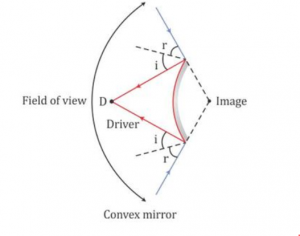### MCQ-7(C) Reflection of Light Selina Revised Concise 9th Physics Solutions

#### Question 1

For an incident ray directed towards centre of curvature of a spherical mirror the reflected ray:

(a) Retraces its path

(b) Passes through the focus

(c) Passes through the pole

(d) Becomes parallel to the principal axis.

Retraces its path

#### Question 2

The image formed by a convex mirror is

(a) Erect and diminished.

(b) Erect and enlarged.

(c) Inverted and diminished.

(d) Inverted and enlarged.

Erect and diminished

#### Question 3

A real and enlarged image can be obtained by using a

(a) Convex mirror

(b) Plane mirror

(c) Concave mirror

(d) Either convex or plane mirror.

Concave mirror

### NUM-7(C) Reflection of Light Selina Concise 9th Physics Solutions

#### Question 1

The radius of curvature of a convex mirror is 40 cm. Find its focal length.

Focal length = ½ (Radius of curvature)

Or, f = 40/2 = 20 cm

#### Question 2

The focal length of a concave mirror is 10 cm. Find its radius of curvature.

Radius of curvature = 2focal length

Or, R = 2f = 210 = 20 cm

#### Question 3

An object of height 2 cm is placed at a distance 20 cm in front of a concave mirror of focal length 12 cm. Find the position, size and nature of the image by drawing.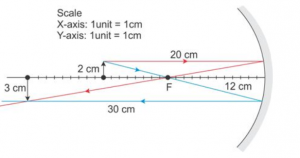The image is 30 cm in front of the mirror, 3 cm high, real, inverted and magnified.

#### Question 4

An object is placed at 4 cm distance in front of a concave mirror of radius of curvature 24 cm. Find the position of image by drawing. Is the image magnified?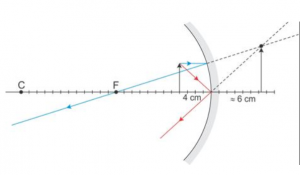The image is 6 cm behind the mirror.

Yes the image is magnified.

#### Question 5

At what distance from a concave mirror of focal length 25 cm should an object be placed so that the size of image is equal to the size of the object. Draw a ray diagram to show this.

The size of the image is equal to the size of the object if the object is placed at the centre of curvature of a concave mirror.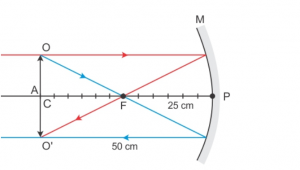Hence, the object should be placed at 50 cm.

#### Question 6

An object 5 cm high is placed at a distance 60 cm in front of a concave mirror of focal length 10 cm. Find the position and size of the image by drawing.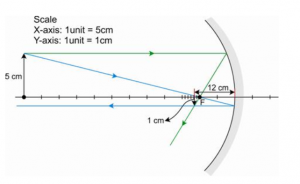The position of the object is 12 cm in front of the mirror.

Its size is 1 cm.

#### Question 7

A point light source is kept in front of a convex mirror at a distance of 40 cm. The focal length of the mirror is 40 cm. Find the position of image by drawing.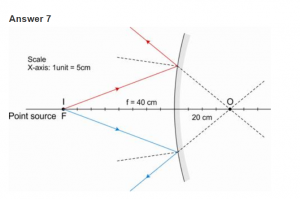The image is behind the mirror at a distance 20 cm

#### Question 8.

When an object of height 1 cm is kept at a distance 4 cm from a concave mirror, its erect image of height 1.5 cm is formed at a distance 6 cm behind the mirror. Find the focal length of mirror, by drawing.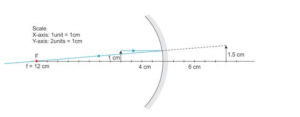A ray passing parallel to the principal axis passes through the focal point after reflection. Hence, the focal length is 12 cm.

#### Question 9

An object of length 4 cm is placed in front of a concave mirror at distance 30 cm. The focal length of mirror is 15 cm.

(a) Where will the image form?

(b) What will be the length of image?

#### Question 10

A concave mirror forms a real image of an object placed in front of it at a distance 30 cm, of size three times the size of object. Find (a) the focal length of mirror (b) position of image.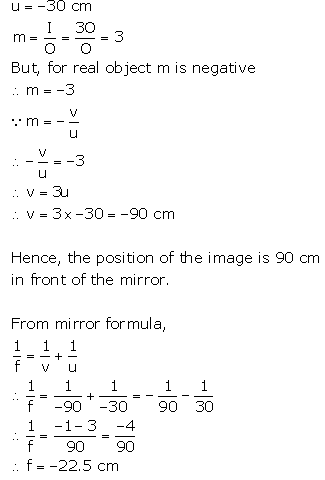#### Question 11

A concave mirror forms a virtual image of size twice that of the object placed at a distance 5 cm from it.

Find : (a) the focal length of the mirror (b) position of image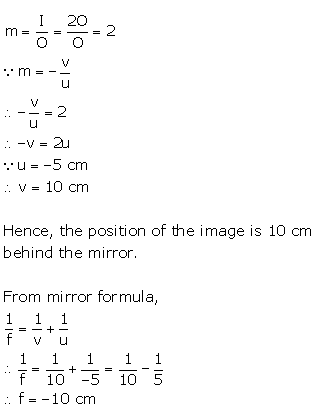#### Question 12

The image formed by a convex mirror is of size one third the size of object. How are u and v related?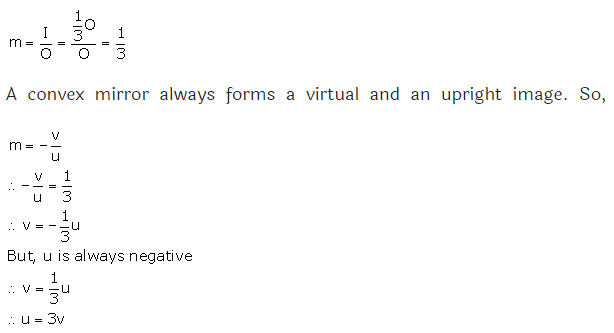#### Question 13

The erect image formed by a concave mirror is of size double the size of object. How are u and v related?

Magnification is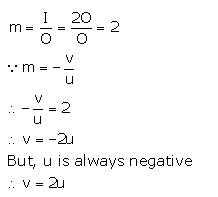#### Question 14

The magnification for a mirror is -3. How are u and v related?

Magnification of a mirror is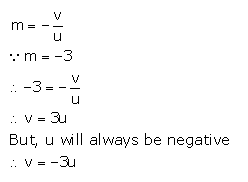Thanks

RELATED ARTICLES